Question

# A buffered solution containing dissolved aniline, C6H5NH2, and aniline hydrochloride, C6H5NH3Cl, has a pH of 5.58...

A buffered solution containing dissolved aniline, C6H5NH2,

and aniline hydrochloride, C6H5NH3Cl, has a pH of 5.58

A. Determine the concentration of C6H5NH+3 in the solution if the concentration of C6H5NH2 is 0.275 M. The p?b of aniline is 9.13.

A buffered solution containing dissolved aniline, C6H5NH2,

and aniline hydrochloride, C6H5NH3Cl, has a pH of 5.58

.

A. Determine the concentration of C6H5NH+3

in the solution if the concentration of C6H5NH2 is 0.275 M. The p?b of aniline is 9.13.

B. Calculate the change in pH of the solution, ΔpH,

if 0.398 g NaOH is added to the buffer for a final volume of 1.65 L. Assume that any contribution of NaOH to the volume is negligible.

(A.) concentration of C6H5NH3+ = 0.0536 M

(B.) change in pH = 0.061

Explanation

(A.) pKb of aniline = 9.13

pKa of anilinium ion = pKw - pKa

pKa of anilinium ion = 14 - 9.13

pKa of anilinium ion = 4.87

According to Henderson - Hasselbalch equation,

pH = pKa + log([conjugate base] / [weak acid])

pH = pKa + log([C6H5NH2] / [C6H5NH3+])

5.58 = 4.87 + log(0.275 M / [C6H5NH3+])

log(0.275 M / [C6H5NH3+]) = 5.58 - 4.87

log(0.275 M / [C6H5NH3+]) = 0.71

0.275 M / [C6H5NH3+] = 100.71

0.275 M / [C6H5NH3+] = 5.13

[C6H5NH3+] = 0.275 M / 5.13

[C6H5NH3+] = 0.0536 M

#### Earn Coins

Coins can be redeemed for fabulous gifts.

Similar Homework Help Questions
• ### A buffered solution containing dissolved aniline, C6H5NH2, and aniline hydrochloride, C6H5NH3Cl, has a pH of 5.65....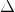A buffered solution containing dissolved aniline, C6H5NH2, and aniline hydrochloride, C6H5NH3Cl, has a pH of 5.65. a) Determine the concentration of C6H5NH3 in the solution if the concentration of C6H5NH2 is 0.230 M. The pKb of aniline is 9.13. [C6H5NH3+] = ______M b)Calculate the change in pH of the solution, ?pH, if 0.386 g NaOH is added to the buffer for a final volume of 1.45 L. Assume that any contribution of NaOH to the volume is negligible. pH =...

• ### A buffered solution containing dissolved aniline, C_6H_5NH_2. and aniline hydrochloride, C_6H_5NH_3CI, has a pH of 5.33....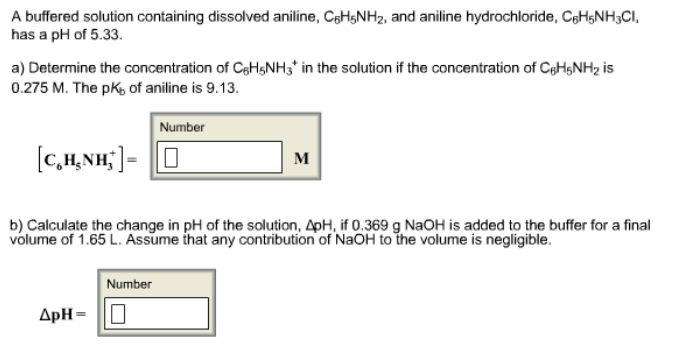A buffered solution containing dissolved aniline, C_6H_5NH_2. and aniline hydrochloride, C_6H_5NH_3CI, has a pH of 5.33. Determine the concentration of C_6H_5NH_3+ in the solution if the concentration of C_6H_5NH_2 is 0.275 M. The pK_b, of aniline is 9.13. Calculate the change in pH of the solution, Delta pH, if 0.369 g NaOH is added to the buffer for a final volume of 1.65 L. Assume that any contribution of NaOH to the volume is negligible.

• ### A buffered solution containing dissolved aniline, CgHNH2, and aniline hydrochloride, CHNH3 Cl, has a pH of...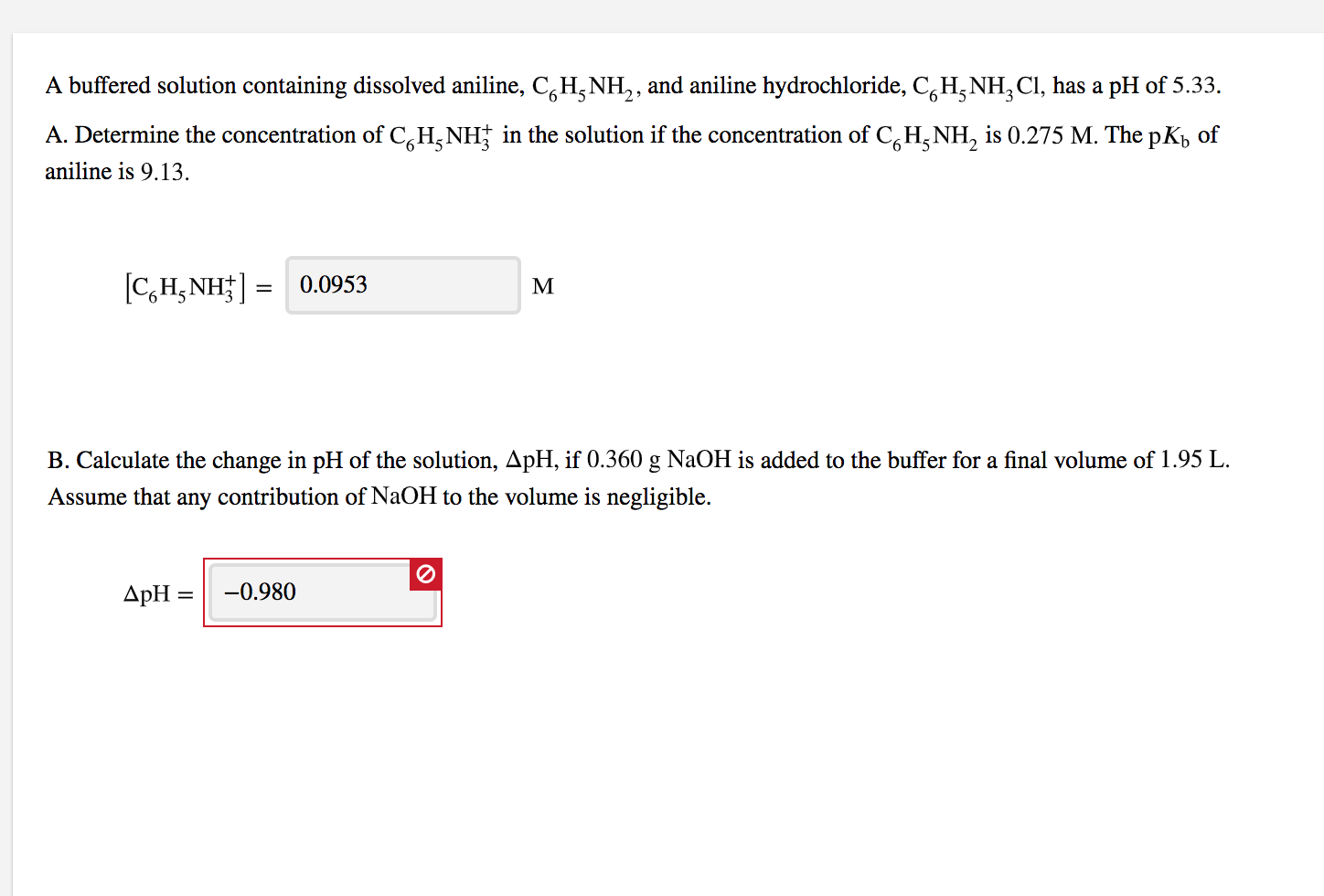A buffered solution containing dissolved aniline, CgHNH2, and aniline hydrochloride, CHNH3 Cl, has a pH of 5.33. A. Determine the concentration of CHNHt in the solution if the concentration of CHNH, is 0.275 M. The pKb of aniline is 9.13. C,HNH 0.0953 B. Calculate the change in pH of the solution, ApH, if 0.360 g NaOH is added to the buffer for a final volume of 1.95 L. Assume that any contribution of N2OH to the volume is negligible -0.980...

• ### A buffered solution containing dissolved aniline, CH,NH, and aniline hydrochloride, CH NH, CI, has a pH...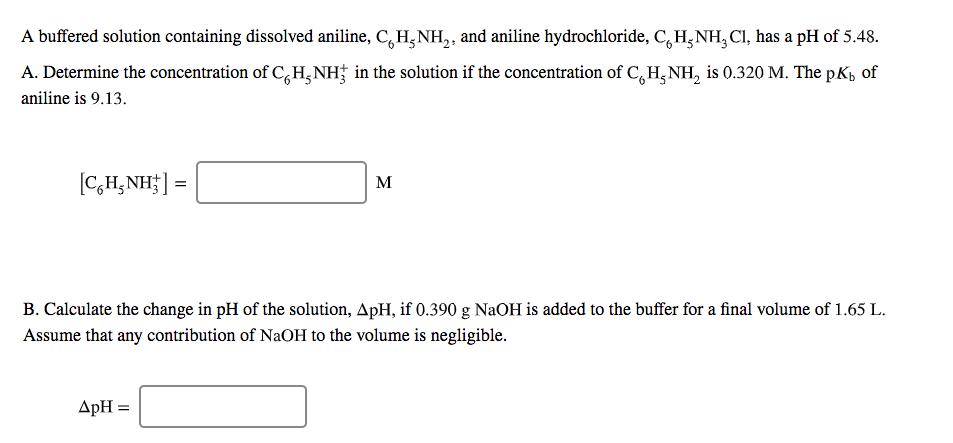A buffered solution containing dissolved aniline, CH,NH, and aniline hydrochloride, CH NH, CI, has a pH of 5.48. A. Determine the concentration of CH NH in the solution if the concentration of C, H,NH, is 0.320 M. The pKb of aniline is 9.13. [C,H, NH] = B. Calculate the change in pH of the solution, ApH, if 0.390 g NaOH is added to the buffer for a final volume of 1.65 L. Assume that any contribution of NaOH to the...

• ### A buffered solution containing dissolved aniline, CH, NH,, and aniline hydrochloride, CH NH, CI, has a...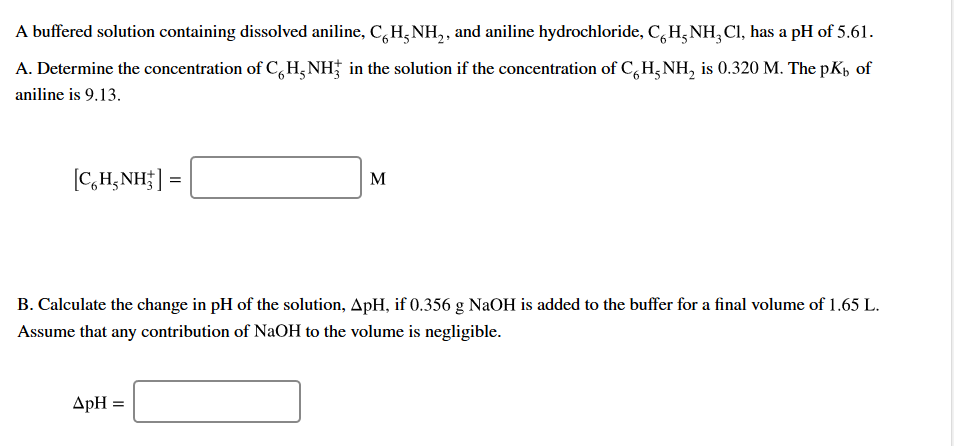A buffered solution containing dissolved aniline, CH, NH,, and aniline hydrochloride, CH NH, CI, has a pH of 5.61. A. Determine the concentration of C H NH in the solution if the concentration of C, H, NH, is 0.320 M. The pKb of aniline is 9.13. [CH, NH] = B. Calculate the change in pH of the solution, ApH, if 0.356 g NaOH is added to the buffer for a final volume of 1.65 L. Assume that any contribution of...

• ### A buffered solution containing dissolved aniline, CH-NH2, and aniline hydrochloride, CH3NH, CI, has a pH of...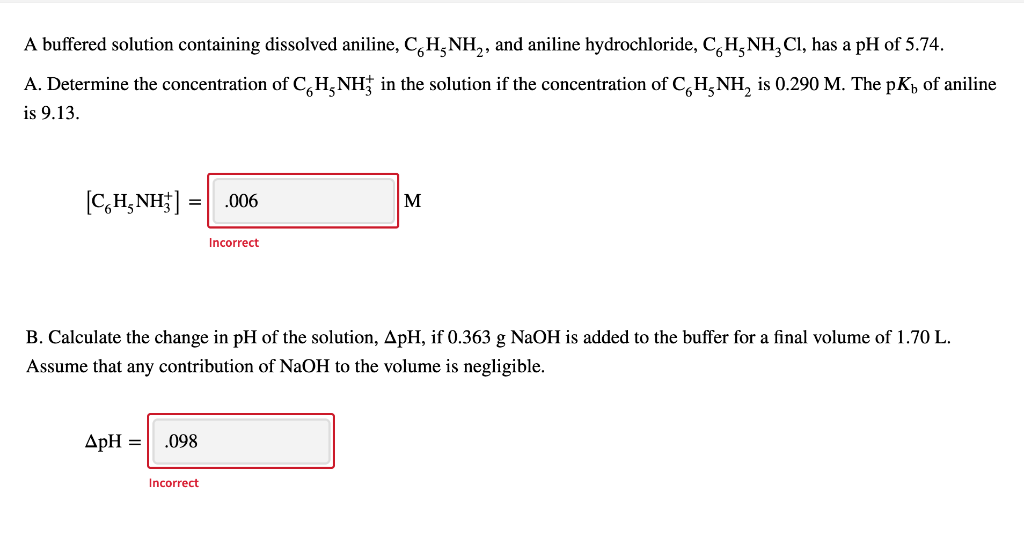A buffered solution containing dissolved aniline, CH-NH2, and aniline hydrochloride, CH3NH, CI, has a pH of 5.74. A. Determine the concentration of CH NH4 in the solution if the concentration of CH_NH, is 0.290 M. The pK) of aniline is 9.13. [CH, NH] : .006 M Incorrect B. Calculate the change in pH of the solution, ApH, if 0.363 g NaOH is added to the buffer for a final volume of 1.70 L. Assume that any contribution of NaOH to...

• ### A buffered solution containing dissolved aniline, CH NH, and aniline hydrochloride, CH-NH, CI, has a pH...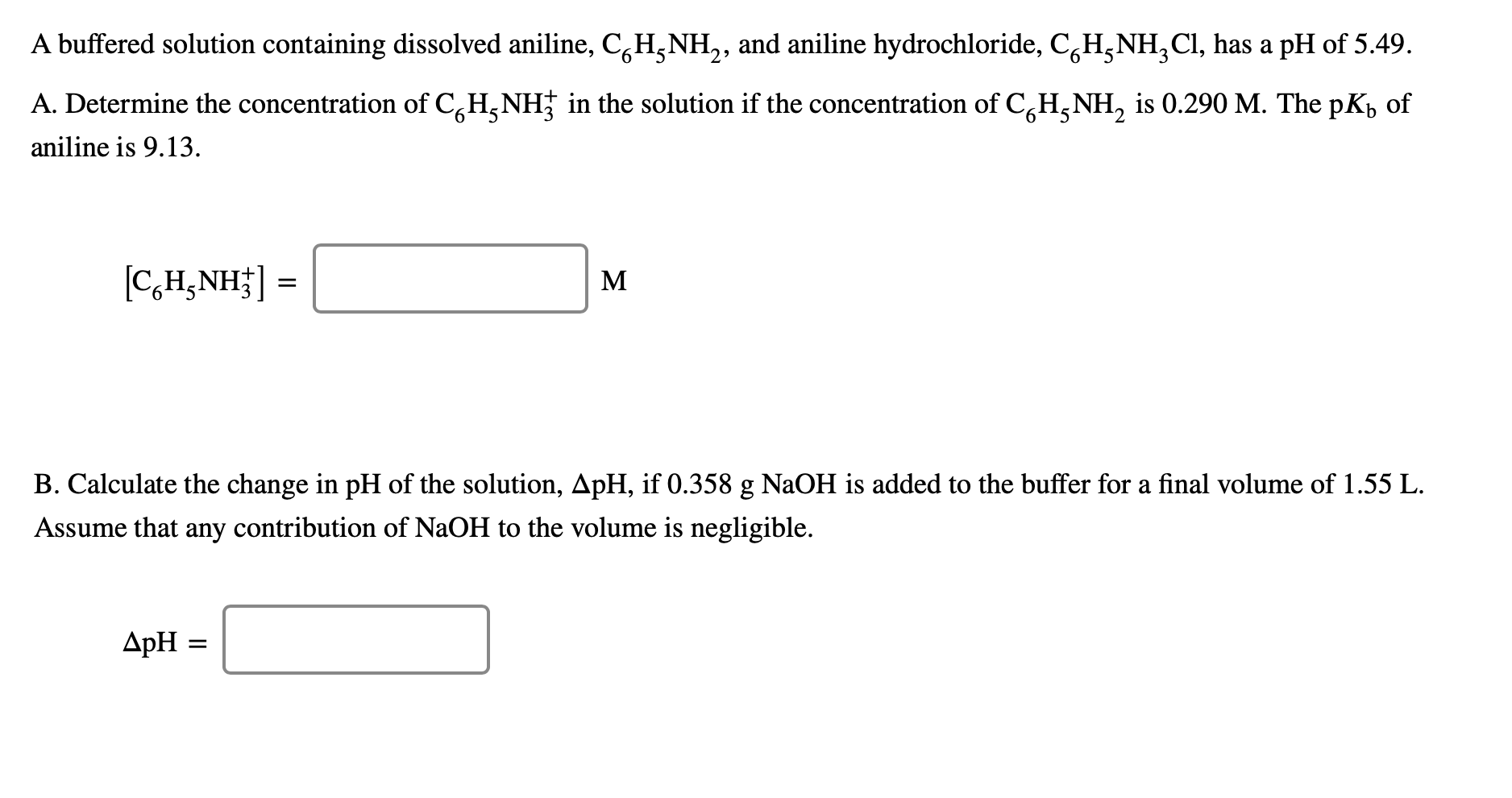A buffered solution containing dissolved aniline, CH NH, and aniline hydrochloride, CH-NH, CI, has a pH of 5.49. A. Determine the concentration of CGH NH; in the solution if the concentration of CoH_NH, is 0.290 M. The pKy of aniline is 9.13. [C,H,NH] = M B. Calculate the change in pH of the solution, ApH, if 0.358 g NaOH is added to the buffer for a final volume of 1.55 L. Assume that any contribution of NaOH to the volume...

• ### solution containing dissolved aniline, C_6H_5NH_2, and aniline hydrochloride, C_6H_5NH_3CI, has a pH of 5.71. Determine the...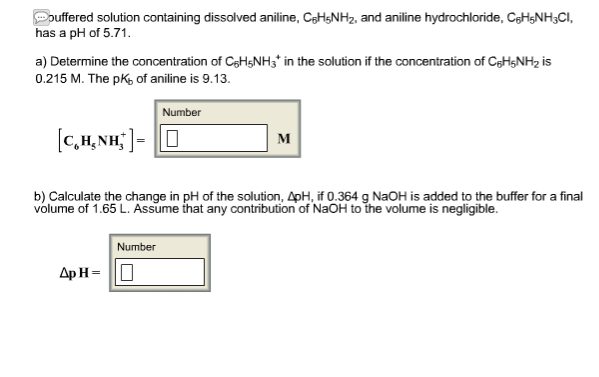solution containing dissolved aniline, C_6H_5NH_2, and aniline hydrochloride, C_6H_5NH_3CI, has a pH of 5.71. Determine the concentration of C_6H_5NH_3^+ in the solution if the concentration of C_6H)_5NH_2 is 0.215 M. The pK_b of aniline is 9.13 [C_6H_5NH_3^+ ] = Calculate the change in delta pH of the solution, delta pH, if0.364 g NaOH is added to the buffer for a final volume of 1.65 L. Assume that any contribution of NaOH to the volume is negligible. Delta p H =

• ### A buffered solution containing dissolved aniline, C H NH2, and aniline hydrochloride, CH-NH, CI, has a...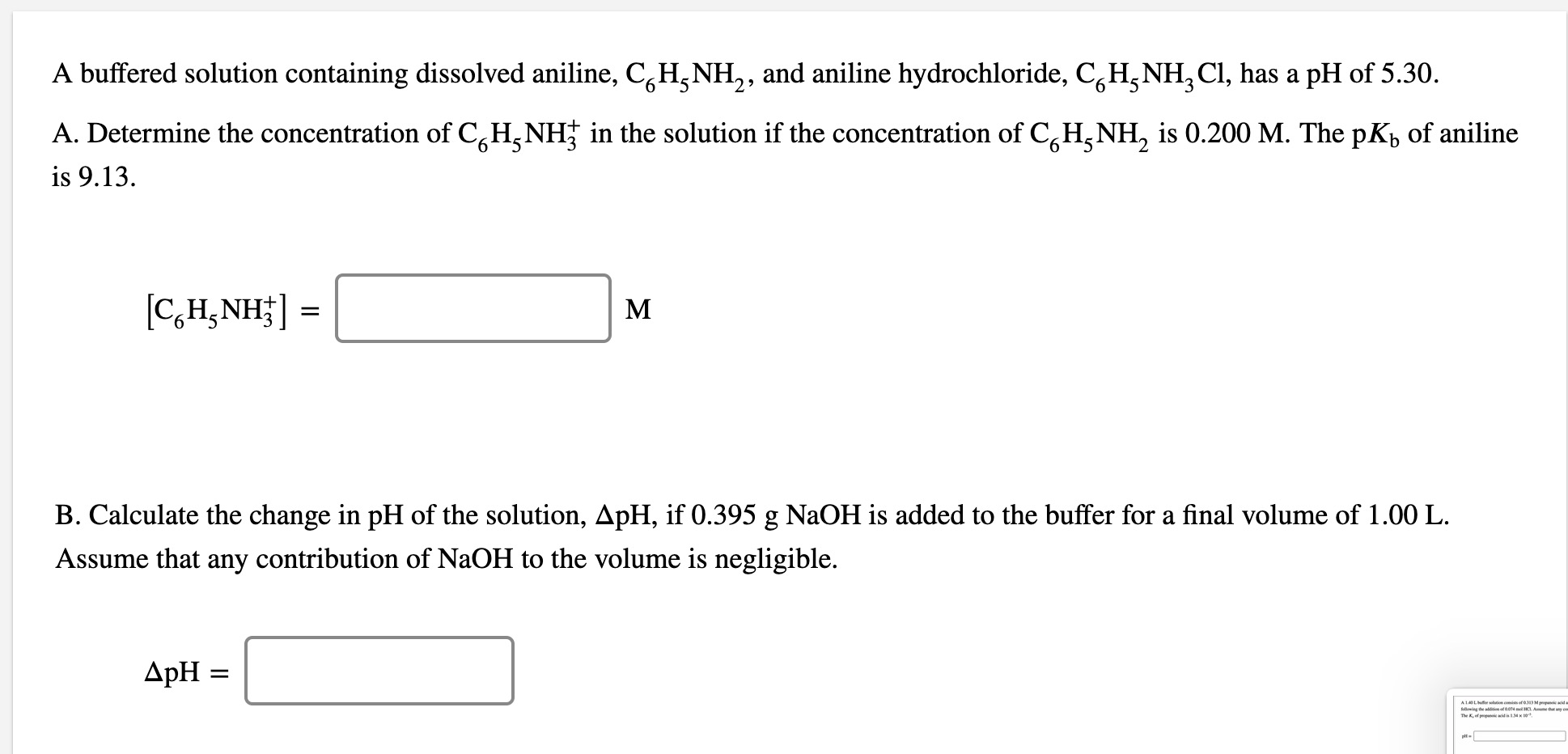A buffered solution containing dissolved aniline, C H NH2, and aniline hydrochloride, CH-NH, CI, has a pH of 5.30. A. Determine the concentration of CGH NH4 in the solution if the concentration of C, H,NH, is 0.200 M. The pKy of aniline is 9.13. [CH NH}] M B. Calculate the change in pH of the solution, ApH, if 0.395 g NaOH is added to the buffer for a final volume of 1.00 L. Assume that any contribution of NaOH to...

• ### Determine the pH of a 2.0 x10^-4 M solution of aniline hydrochloride (C6H5NH3Cl). Kb aniline (C6H5NH2)...

Determine the pH of a 2.0 x10^-4 M solution of aniline hydrochloride (C6H5NH3Cl). Kb aniline (C6H5NH2) = 3.98 x10^-10